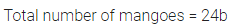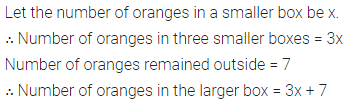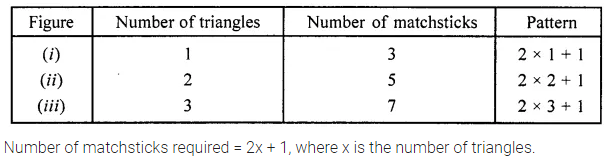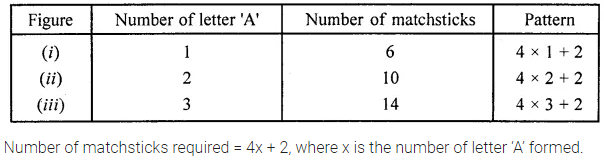# ML Aggarwal Class 6 Solutions for ICSE Maths Chapter 9 Algebra Ex 9.1

## ML Aggarwal Class 6 Solutions for ICSE Maths Chapter 9 Algebra Ex 9.1

Question 1.
Find the rule which gives the number of matchsticks required to make the following matchsticks patterns. Use a variable to write the rule.Solution:Question 2.
If there are 24 mangoes in a box, how will you write the number of mangoes in terms of the number of boxes? (use b for the number of boxes.)
Solution:Question 3.
Anuradha is drawing a dot Rangoli (a beautiful pattern of lines joining dots). She has 8 dots in a row. How many dots will her Rangoli have for r rows? How many dots are there if there are 12 rows?
Solution:Question 4.
Anu and Meenu are sisters. Anu is 5 years younger than Meenu. Can you write Anu’s age in terms of Meenu’s age? Take Meenu’s age as x years.
Solution:Question 5.
Oranges are to be transferred from larger boxes to smaller boxes. When a larger box is emptied, the oranges from it fill 3 samller boxes and still 7 oranges are left. If the number of oranges in a small box is taken to be x, then what is the number of oranges in the larger box?
Solution:Question 6.
Harsha’s score in Mathematics is 15 more than three-fourth of her score in Science. If she scores x marks in Science, find her score in Mathematics?
Solution:Question 7.
Look at the following matchstick pattern of equilateral triangles. The triangles are not separate. Two neighbouring triangles have a common matchstick. Observe the pattern and find the rule that gives the number of matchsticks.Solution:Question 8.
Look at the following matchstick pattern of letter A. The A’s are not separate. Two neighbouring A’s have two common matchsticks. Observe the pattern and find the rule that gives the number of matchsticks.Solution: